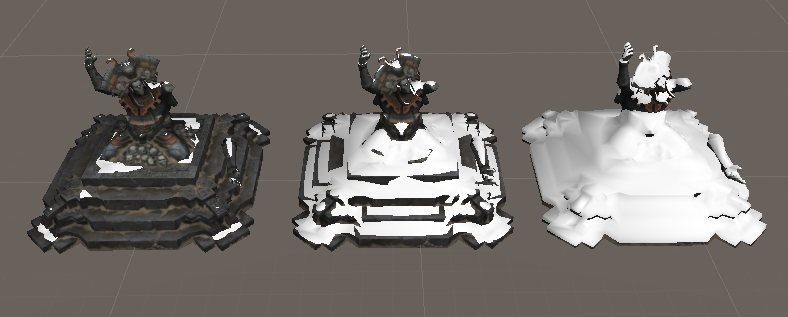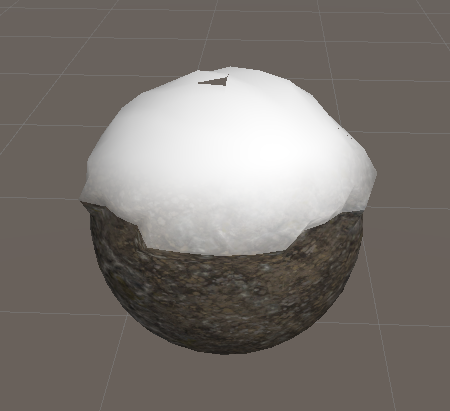```v2f vert (appdata v)
{
...
float4 uvNoisetex=tex2Dlod(_VertNoiseTex,float4(TRANSFORM_TEX(o.uvNoise.xy,_VertNoiseTex),0,0));
if(dot(wN,_SnowDirection)>lerp(1,-1,_Snow)){
v.vertex.xyz+=uvNoisetex.rgb* _VertOut*v.normal;
v.vertex.y+=_VertHeight+_VertOut;
}
...
}```

```fixed4 frag (v2f i) : SV_Target
{
//法线与假定的下雪方向做点积计算
float edge=dot(N,_SnowDirection);
if(edge>lerp(1,-1,_Snow)){
//边缘做一个宽度的渐变
float sm=smoothstep(0,_SnowEdgeOut,edge);
//积雪边缘的过渡
fixed3 xue=lerp(col.rgb,_SnowColor.rgb,sm);
//积雪的颜色加了个透明
col.rgb=lerp(col.rgb,xue,_SnowAlpha);
}
}```